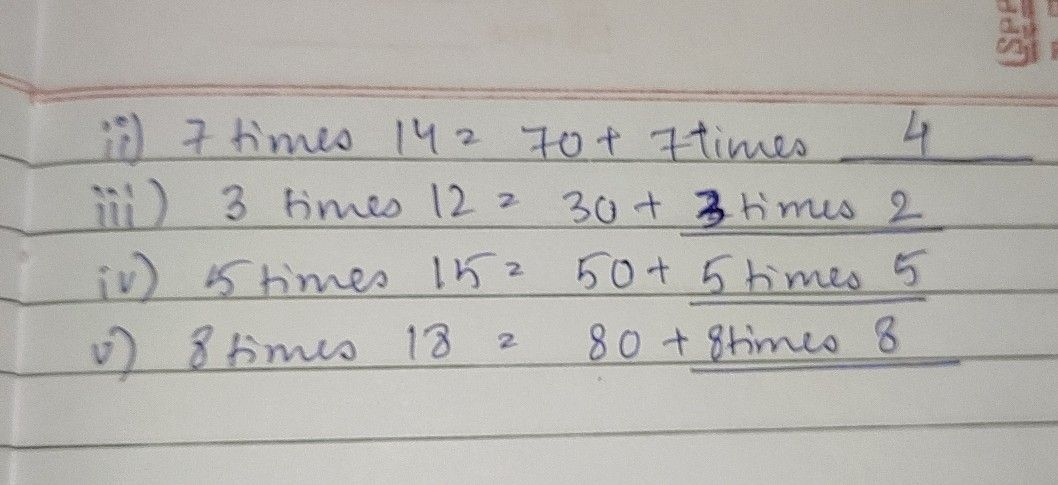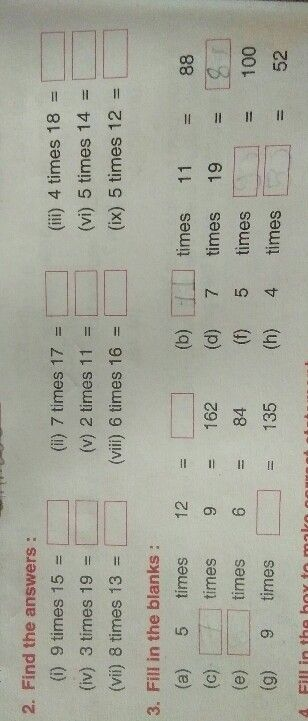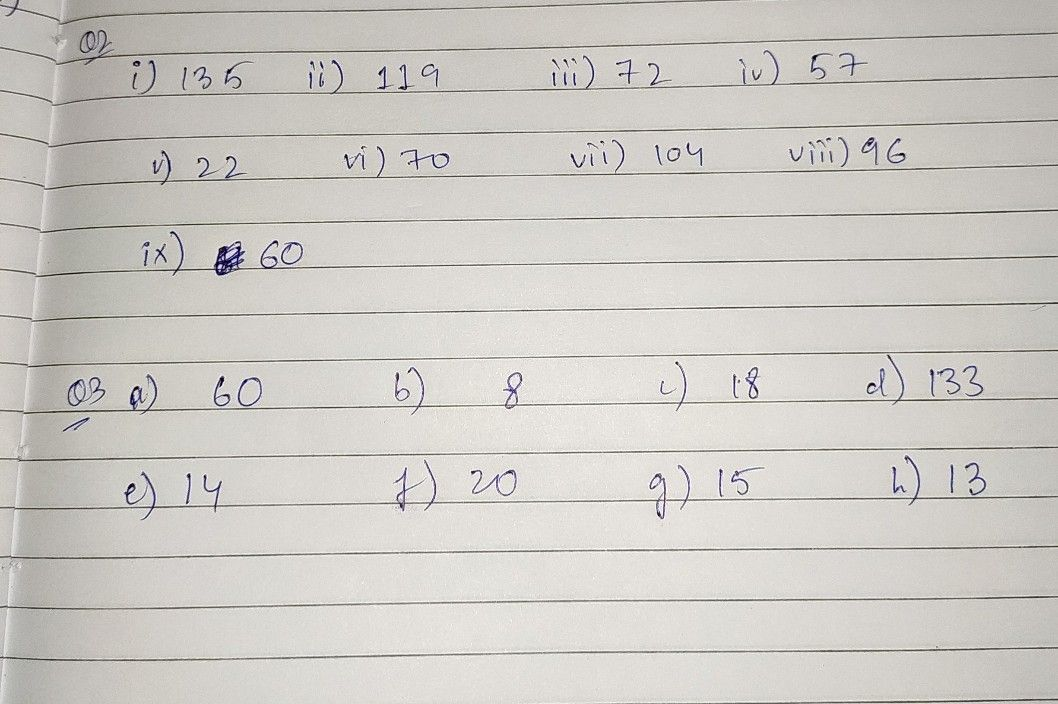Symbol
Problem6 times $16=6$ times $10+6$ times $\vec{p}$ times $14=70+7$ times 3times $12=30+$ $5$ 5 times $15=50+$ 0 $8$ 8 times $18=80+$
Other
Question content
6times 16 = 6times 10+6times
SolutionQanda teacher - gaurav sir